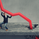# Universal Time Frame - Day Trading Italia

803 views
803
```study("Universal Time Frame", shorttitle = "uniTF", overlay=true)
higherRes = input("15", type=resolution)
upperBound = input(high, type=source)
lowerBound = input(low, type=source)

isNewBar(res) =>
t = time(res)
change(t) != 0 ? 1 : 0

isnb = isNewBar(higherRes)

ub = isnb ? na : security(tickerid, higherRes, upperBound)
lb = isnb ? na : security(tickerid, higherRes, lowerBound)

p1 = plot(ub, title="upperBound", color= lime, style=linebr, join=false, transp=70)
p2 = plot(lb, title="lowerBound", color= lime, style=linebr, join=false, transp=70)

fill(p1, p2, color=lime, transp=70)
```Hi, could you please add an option to show the box from input time range? for example from 1am to 4:AM